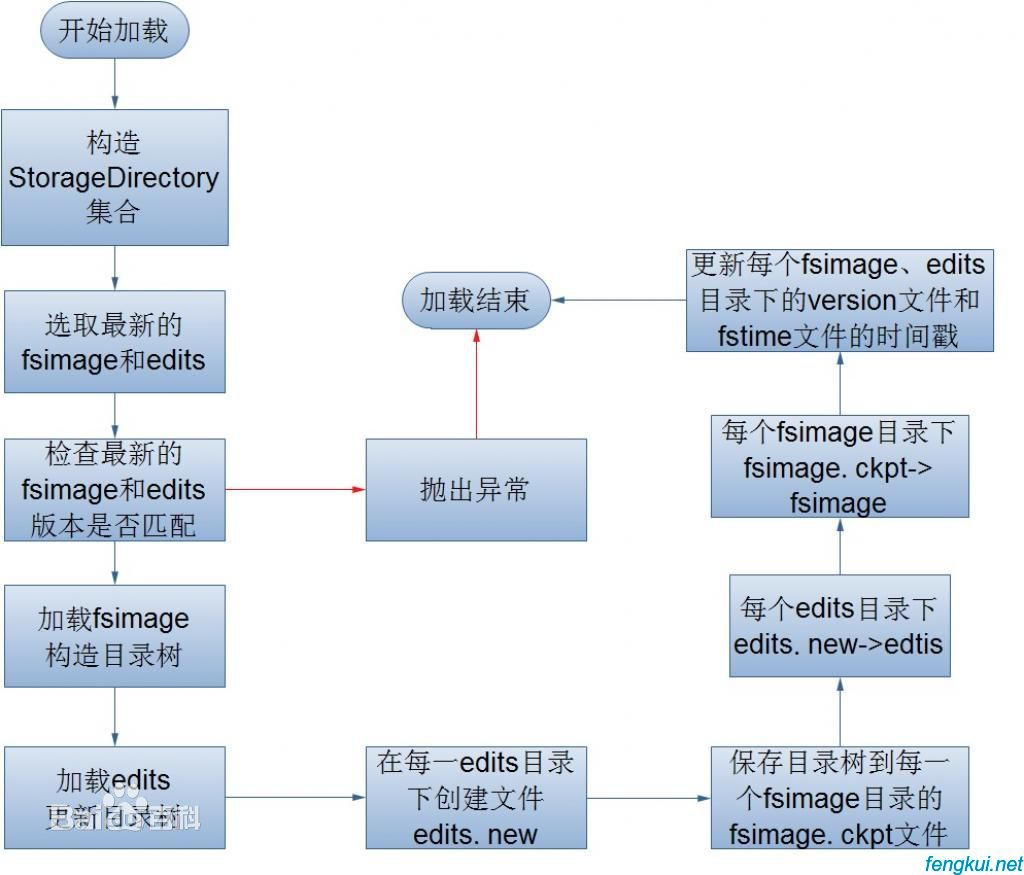# PHP 命名空间(namespace)

PHP 命名空间(namespace)是在PHP 5.3中加入的，如果你学过C#和Java，那命名空间就不算什么新事物。 不过在PHP当中还是有着相当重要的意义。

PHP 命名空间可以解决以下两类问题：

1. 用户编写的代码与PHP内部的类/函数/常量或第三方类/函数/常量之间的名字冲突。
2. 为很长的标识符名称(通常是为了缓解第一类问题而定义的)创建一个别名（或简短）的名称，提高源代码的可读性。
• -

``````<?php
// 定义代码在 'MyProject' 命名空间中
namespace MyProject;
// ... 代码 ...``````

``````<?php
namespace MyProject;
const CONNECT_OK = 1;
class Connection { /* ... */ }
function connect() { /* ... */  }
namespace AnotherProject;
const CONNECT_OK = 1;
class Connection { /* ... */ }
function connect() { /* ... */  }
?>``````

``````<?php
namespace MyProject {
const CONNECT_OK = 1;
class Connection { /* ... */ }
function connect() { /* ... */  }
}
namespace AnotherProject {
const CONNECT_OK = 1;
class Connection { /* ... */ }
function connect() { /* ... */  }
}
?>``````

``````<?php
namespace MyProject {
const CONNECT_OK = 1;
class Connection { /* ... */ }
function connect() { /* ... */  }
}
namespace { // 全局代码
session_start();
\$a = MyProject\connect();
echo MyProject\Connection::start();
}
?>``````

``````<?php
declare(encoding='UTF-8'); //定义多个命名空间和不包含在命名空间中的代码
namespace MyProject {
const CONNECT_OK = 1;
class Connection { /* ... */ }
function connect() { /* ... */  }
}
namespace { // 全局代码
session_start();
\$a = MyProject\connect();
echo MyProject\Connection::start();
}
?>``````

``````<html>
<?php
namespace MyProject; // 命名空间前出现了“<html>” 会致命错误 - 命名空间必须是程序脚本的第一条语句
?>``````
• -

``````<?php
namespace MyProject\Sub\Level;  //声明分层次的单个命名空间
const CONNECT_OK = 1;
class Connection { /* ... */ }
function Connect() { /* ... */  }
?>``````

• -

PHP 命名空间中的类名可以通过三种方式引用：

1. 非限定名称，或不包含前缀的类名称，例如 \$a=new foo(); 或 foo::staticmethod();。如果当前命名空间是 currentnamespace，foo 将被解析为 currentnamespace\foo。如果使用 foo 的代码是全局的，不包含在任何命名空间中的代码，则 foo 会被解析为foo。 警告：如果命名空间中的函数或常量未定义，则该非限定的函数名称或常量名称会被解析为全局函数名称或常量名称。
2. 限定名称,或包含前缀的名称，例如 \$a = new subnamespace\foo(); 或 subnamespace\foo::staticmethod();。如果当前的命名空间是 currentnamespace，则 foo 会被解析为 currentnamespace\subnamespace\foo。如果使用 foo 的代码是全局的，不包含在任何命名空间中的代码，foo 会被解析为subnamespace\foo。
3. 完全限定名称，或包含了全局前缀操作符的名称，例如， \$a = new \currentnamespace\foo(); 或 \currentnamespace\foo::staticmethod();。在这种情况下，foo 总是被解析为代码中的文字名(literal name)currentnamespace\foo。

file1.php 文件代码

``````<?php
namespace Foo\Bar\subnamespace;
const FOO = 1;
function foo() {}
class foo
{
static function staticmethod() {}
}
?>``````

file2.php 文件代码

``````<?php
namespace Foo\Bar;
include 'file1.php';
const FOO = 2;
function foo() {}
class foo
{
static function staticmethod() {}
}
/* 非限定名称 */
foo(); // 解析为 Foo\Bar\foo resolves to function Foo\Bar\foo
foo::staticmethod(); // 解析为类 Foo\Bar\foo的静态方法staticmethod。resolves to class Foo\Bar\foo, method staticmethod
echo FOO; // resolves to constant Foo\Bar\FOO
/* 限定名称 */
subnamespace\foo(); // 解析为函数 Foo\Bar\subnamespace\foo
subnamespace\foo::staticmethod(); // 解析为类 Foo\Bar\subnamespace\foo,
// 以及类的方法 staticmethod
echo subnamespace\FOO; // 解析为常量 Foo\Bar\subnamespace\FOO

/* 完全限定名称 */
\Foo\Bar\foo(); // 解析为函数 Foo\Bar\foo
\Foo\Bar\foo::staticmethod(); // 解析为类 Foo\Bar\foo, 以及类的方法 staticmethod
echo \Foo\Bar\FOO; // 解析为常量 Foo\Bar\FOO
?>``````

``````<?php
namespace Foo;
function strlen() {}
const INI_ALL = 3;
class Exception {}
\$a = \strlen('hi'); // 调用全局函数strlen
\$b = \INI_ALL; // 访问全局常量 INI_ALL
\$c = new \Exception('error'); // 实例化全局类 Exception
?>``````

• -

PHP 命名空间的实现受到其语言自身的动态特征的影响。因此，如果要将下面的代码转换到命名空间中，动态访问元素。

example1.php 文件代码：

``````<?php
class classname
{
function __construct()
{
echo __METHOD__,"\n";
}
}
function funcname()
{
echo __FUNCTION__,"\n";
}
const constname = "global";
\$a = 'classname';
\$obj = new \$a; // prints classname::__construct
\$b = 'funcname';
\$b(); // prints funcname
echo constant('constname'), "\n"; // prints global
?>``````

``````<?php
namespace namespacename;
class classname
{
function __construct()
{
echo __METHOD__,"\n";
}
}
function funcname()
{
echo __FUNCTION__,"\n";
}
const constname = "namespaced";
include 'example1.php';
\$a = 'classname';
\$obj = new \$a; // prints classname::__construct
\$b = 'funcname';
\$b(); // prints funcname
echo constant('constname'), "\n"; // prints global
/* note that if using double quotes, "\\namespacename\\classname" must be used */
\$a = '\namespacename\classname';
\$obj = new \$a; // prints namespacename\classname::__construct
\$a = 'namespacename\classname';
\$obj = new \$a; // also prints namespacename\classname::__construct
\$b = 'namespacename\funcname';
\$b(); // prints namespacename\funcname
\$b = '\namespacename\funcname';
\$b(); // also prints namespacename\funcname
echo constant('\namespacename\constname'), "\n"; // prints namespaced
echo constant('namespacename\constname'), "\n"; // also prints namespaced
?>``````
• -

## namespace关键字和__NAMESPACE__常量

PHP支持两种抽象的访问当前命名空间内部元素的方法，__NAMESPACE__ 魔术常量和namespace关键字。

NAMESPACE 示例, 在命名空间中的代码

``````<?php
namespace MyProject;
echo '"', __NAMESPACE__, '"'; // 输出 "MyProject"
?>``````

__NAMESPACE__ 示例，全局代码

``````<?php
echo '"', __NAMESPACE__, '"'; // 输出 ""
?>``````

``````<?php
namespace MyProject;
function get(\$classname)
{
\$a = __NAMESPACE__ . '\\' . \$classname;
return new \$a;
}
?>``````

namespace操作符，命名空间中的代码

``````<?php
namespace MyProject;
use blah\blah as mine; // see "Using namespaces: importing/aliasing"
blah\mine(); // calls function blah\blah\mine()
namespace\blah\mine(); // calls function MyProject\blah\mine()
namespace\func(); // calls function MyProject\func()
namespace\sub\func(); // calls function MyProject\sub\func()
namespace\cname::method(); // calls static method "method" of class MyProject\cname
\$a = new namespace\sub\cname(); // instantiates object of class MyProject\sub\cname
\$b = namespace\CONSTANT; // assigns value of constant MyProject\CONSTANT to \$b
?>``````

namespace操作符, 全局代码

``````<?php
namespace\func(); // calls function func()
namespace\sub\func(); // calls function sub\func()
namespace\cname::method(); // calls static method "method" of class cname
\$a = new namespace\sub\cname(); // instantiates object of class sub\cname
\$b = namespace\CONSTANT; // assigns value of constant CONSTANT to \$b
?>``````

• -

PHP 命名空间支持 有两种使用别名或导入方式：为类名称使用别名，或为命名空间名称使用别名。

1、使用use操作符导入/使用别名

``````<?php
namespace foo;
use My\Full\Classname as Another;
// 下面的例子与 use My\Full\NSname as NSname 相同
use My\Full\NSname;
// 导入一个全局类
use \ArrayObject;
\$obj = new namespace\Another; // 实例化 foo\Another 对象
\$obj = new Another; // 实例化 My\Full\Classname 对象
NSname\subns\func(); // 调用函数 My\Full\NSname\subns\func
\$a = new ArrayObject(array(1)); // 实例化 ArrayObject 对象
// 如果不使用 "use \ArrayObject" ，则实例化一个 foo\ArrayObject 对象
?>``````

2、 一行中包含多个use语句

``````<?php
use My\Full\Classname as Another, My\Full\NSname;
\$obj = new Another; // 实例化 My\Full\Classname 对象
NSname\subns\func(); // 调用函数 My\Full\NSname\subns\func
?>``````

3、导入和动态名称

``````<?php
use My\Full\Classname as Another, My\Full\NSname;
\$obj = new Another; // 实例化一个 My\Full\Classname 对象
\$a = 'Another';
\$obj = new \$a;      // 实际化一个 Another 对象
?>``````

4、导入和完全限定名称

``````<?php
use My\Full\Classname as Another, My\Full\NSname;
\$obj = new Another; // instantiates object of class My\Full\Classname
\$obj = new \Another; // instantiates object of class Another
\$obj = new Another\thing; // instantiates object of class My\Full\Classname\thing
\$obj = new \Another\thing; // instantiates object of class Another\thing
?>``````

• -

1、在命名空间中访问全局类

``````<?php
namespace A\B\C;
class Exception extends \Exception {}
\$a = new Exception('hi'); // \$a 是类 A\B\C\Exception 的一个对象
\$b = new \Exception('hi'); // \$b 是类 Exception 的一个对象
\$c = new ArrayObject; // 致命错误, 找不到 A\B\C\ArrayObject 类
?>``````

2、 命名空间中后备的全局函数/常量

``````<?php
namespace A\B\C;
const E_ERROR = 45;
function strlen(\$str)
{
return \strlen(\$str) - 1;
}
echo E_ERROR, "\n"; // 输出 "45"
echo INI_ALL, "\n"; // 输出 "7" - 使用全局常量 INI_ALL
echo strlen('hi'), "\n"; // 输出 "1"
if (is_array('hi')) { // 输出 "is not array"
echo "is array\n";
} else {
echo "is not array\n";
}
?>``````

• -

``````<?php
namespace A\B\C;
/* 这个函数是 A\B\C\fopen */
function fopen() {
/* ... */
\$f = \fopen(...); // 调用全局的fopen函数
return \$f;
}
?>``````

• -

``````<?php
namespace A;
use B\D, C\E as F;
// 函数调用
foo();      // 首先尝试调用定义在命名空间"A"中的函数foo()
// 再尝试调用全局函数 "foo"
\foo();     // 调用全局空间函数 "foo"
my\foo();   // 调用定义在命名空间"A\my"中函数 "foo"
F();        // 首先尝试调用定义在命名空间"A"中的函数 "F"
// 再尝试调用全局函数 "F"
// 类引用
new B();    // 创建命名空间 "A" 中定义的类 "B" 的一个对象
// 如果未找到，则尝试自动装载类 "A\B"
new D();    // 使用导入规则，创建命名空间 "B" 中定义的类 "D" 的一个对象
// 如果未找到，则尝试自动装载类 "B\D"
new F();    // 使用导入规则，创建命名空间 "C" 中定义的类 "E" 的一个对象
// 如果未找到，则尝试自动装载类 "C\E"
new \B();   // 创建定义在全局空间中的类 "B" 的一个对象
// 如果未发现，则尝试自动装载类 "B"
new \D();   // 创建定义在全局空间中的类 "D" 的一个对象
// 如果未发现，则尝试自动装载类 "D"
new \F();   // 创建定义在全局空间中的类 "F" 的一个对象
// 如果未发现，则尝试自动装载类 "F"
// 调用另一个命名空间中的静态方法或命名空间函数
B\foo();    // 调用命名空间 "A\B" 中函数 "foo"
B::foo();   // 调用命名空间 "A" 中定义的类 "B" 的 "foo" 方法
// 如果未找到类 "A\B" ，则尝试自动装载类 "A\B"
D::foo();   // 使用导入规则，调用命名空间 "B" 中定义的类 "D" 的 "foo" 方法
// 如果类 "B\D" 未找到，则尝试自动装载类 "B\D"
\B\foo();   // 调用命名空间 "B" 中的函数 "foo"
\B::foo();  // 调用全局空间中的类 "B" 的 "foo" 方法
// 如果类 "B" 未找到，则尝试自动装载类 "B"
// 当前命名空间中的静态方法或函数
A\B::foo();   // 调用命名空间 "A\A" 中定义的类 "B" 的 "foo" 方法
// 如果类 "A\A\B" 未找到，则尝试自动装载类 "A\A\B"
\A\B::foo();  // 调用命名空间 "A\B" 中定义的类 "B" 的 "foo" 方法
// 如果类 "A\B" 未找到，则尝试自动装载类 "A\B"
?>``````